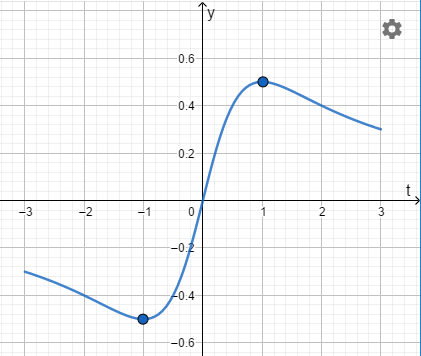# Find the exact global maximum and minimum values (y values) of the function f(t)= \frac...

## Question:

Find the exact global maximum and minimum values ({eq}y {/eq} values) of the function {eq}f(t)= \frac {t}{(1+t^2)} {/eq} on the interval {eq}[-3,3] {/eq}

## Maximum and minimum values

If we have a continuous function with one independent variable and a closed interval, we can find maximum and minimum values with differentiation, because, maximum and minimum values there exist when the first derivative is zero.

The function is:

{eq}\displaystyle \ f(t) \, = \, \frac {t}{1+t^2} \\ {/eq}

The closed interval is:

{eq}\displaystyle [ -3 \; , \; 3 ] \\ {/eq}

Endpoints evaluation is:

{eq}\displaystyle \ f(-3) \, = \, \frac {(-3)}{1+(-3)^2} =-0.3 \\ \; \text{ & } \; \\ \displaystyle \ f(3) \, = \, \frac {(3)}{1+(3)^2} =0.3 \\ {/eq}

Graph:We can find the absolute minimum and maximum value, with the first derivative:

{eq}\displaystyle \ f'(t) \; = \; \left( {t}^{2}+1 \right) ^{-1}-2\,{\frac {{t}^{2}}{ \left( {t}^{2}+1 \right) ^{2}}} \\ \displaystyle \ f'(t) \; = \; -{\frac { \left( t-1 \right) \left( t+1 \right) }{ \left( {t}^{2}+1 \right) ^{2}}} \\ {/eq}

Minimum and maximum value at first derivative zero:

{eq}\displaystyle 0= \ f'(t) \; \; \Rightarrow \; 0= -{\frac { \left( t-1 \right) \left( t+1 \right) }{ \left( {t}^{2}+1 \right) ^{2}}} \; \Leftrightarrow \; x= -1 \; \text{ & } \; x=1 \\ {/eq}

Then, the absolute minimum value is:

{eq}\displaystyle \ f\left( -1 \right) \, = \, \frac {(-1)}{1+(-1)^2} = -0.5 \\ \displaystyle y=-0.5\\ {/eq}

And the absolute minimum point is: {eq}\displaystyle \left( -1 \; , \; -0.5 \right) {/eq}

So, the absolute maximum value is:

{eq}\displaystyle \ f(1) \, = \, \frac {(1)}{1+(1)^2} = 0.5 \\ \displaystyle y=0.5\\ {/eq}

Finally, the absolute maximum point is:

{eq}\displaystyle \left( 1 \; , \; 0.5 \right) \\ {/eq}